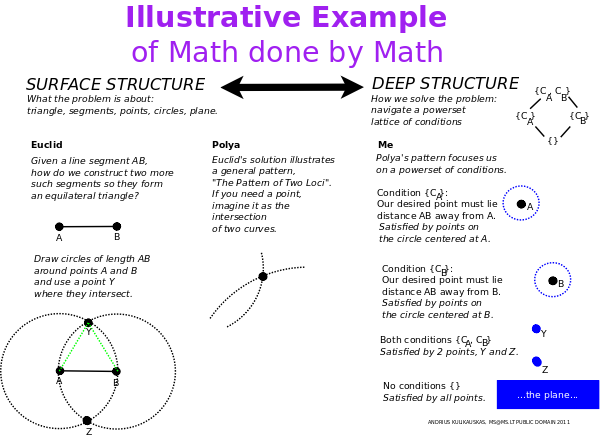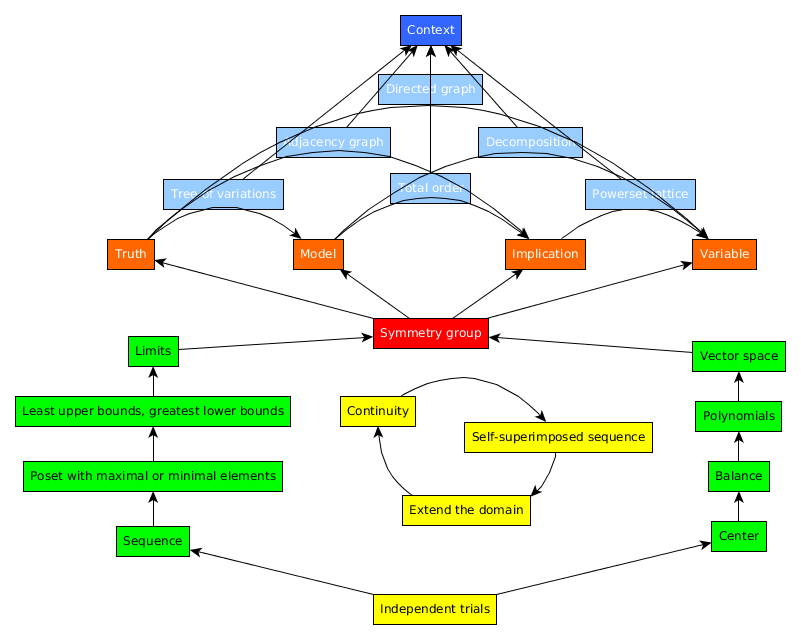# Book: TheMathInOurMinds

Submitted for the European Congress of Analytic Philosophy. Rejected.

The Math In Our Minds By Which We Do Math With Symbols

How can we make sense of the entirety of mathematics? To what extent can we think of mathematical concepts as naturally growing more complex, more concrete and more abstract? By what criteria can we identify the simplest concepts from which all others unfold? In what context can we meaningfully discuss the role of mathematical beauty, intuition, understanding, discovery and community? Can a science of math not only discuss math but also offer practical guidance to help math advance?

Let us distinguish the explicit math which we express with symbols on paper or some other medium and the implicit math which we perform in our minds to discover solutions and apply ideas. How can we describe our experience of our own mathematical activity?

George Polya, in his book, How to Solve It, notes the role of patterns. He considers Euclid's problem of how to construct an equilateral triangle. If we are given the side AB, how do we construct the other two? The solution is a recurring idea which Polya calls the "pattern of two loci". We think of there being two separate conditions. One side must extend a length AB from the point A. Another side must extend a length AB from the point B. We thus draw two circles of radius AB centered at A and B. The points where the two circles intersect are those where we can draw a third point C which satisfies both conditions so that our triangle is equilateral.In our minds, the problem is abstract and simple. Imagine a powerset lattice of conditions. Circle A is one condition, circle B is another condition, and the intersection of A and B satisifies the union of these two conditions. Our minds thus solve the surface problem (constructing a triangle) by considering a simpler, deeper structure (a lattice of conditions). This brings to mind linguist Noah Chomsky's work in syntax and architect Christopher Alexander's work on pattern languages.

The solution took place without supposing a particular axiomatic system. Instead, it made use of a mental construct (the lattice of conditions) that may require practice to consciously master, yet feels native to our cognitive environment, is not restricted to the field of mathematics, and seems pre-mathematical. We may distinguish as cognitively "natural" those math structures which are used by the mind in solving math problems.

I collected such problem solving patterns discussed in Paul Zeitz's book The Art and Craft of Problem Solving and other sources. I sorted almost 200 examples into 24 such patterns. Each distinct pattern makes use of a structure which is familiar to mathematicians and yet is not explicit but mental.

I present the 24 patterns in a system which I argue suggests they are complete.12 of the patterns take place as if prior to an explicit mathematical system. We may think of them as being performed on a generic "mental sheet". We may work on a single mental sheet or a sequence of sheets. This option is itself meaningful as the notion of "independent trials".

Algebra has us work with a single sheet. We may solve a problem by defining its center (origin), by applying parity (as in multiplying numerator and denominator by the same expression), by considering a set (for example, the roots of a polynomial) and a list (a basis of a vector space).

Analysis has us work with an arbitrarily large sequence of sheets, as in applying mathematical induction. We can then focus on maximal or minimal elements, least upper bounds or greatest lower bounds, and also limits.

Algebra and analysis are stitched together by a three-cycle of taking-a-stand, following through and reflecting which is akin to the scientific method of identifying a hypothesis, conducting an experiment and considering the results. In math, the stitching takes place by extending the domain of an existing truth (such as an addition formula), leveraging continuity to find points of interest (such as critical points), and consequently producing a self-superimposed sequence (a generating function).

This activity yields a mathematical system in the form of a symmetry group of transformations. This system then allows us to compare four different metalogics for the "mental sheet" defined by the system and a "mental sheet" of our mind beyond it.

The system suggests a role for 4 different spatial geometries (based on affine paths-forward, projective lines-back-and-forth, conformal angles-around, and symplect oriented-areas-within) which relate to 4 infinite families of polytopes, An (simplexes), Cn (cross polytopes), Bn (hypercubes), Dn (demicubes), whose symmetry groups are also the Weyl groups for the root systems of the classical Lie algebras.

Six network structures are six specifications of one geometry in terms of another. Yet ultimately we need to consider the greater context. If I ask you, what is 10+4? You may say 14, but the answer is 2, because I am thinking about a clock. Which is to say that what we know may be completely irrelevant and plain wrong because ultimately it all depends on context. We have to be willing to go back to the blank sheet.

Parsiųstas iš http://www.ms.lt/sodas/Book/TheMathInOurMinds
Puslapis paskutinį kartą pakeistas 2017 rugsėjo 21 d., 14:40